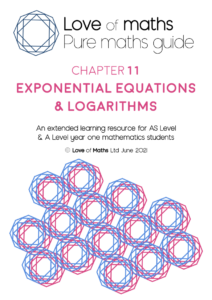Chapter 11 Exponential Equations & Logarithms

£1.00

Chapter 11 – Exponential Equations & Logarithms

Description

Love of Maths – Pure Maths Guide

Chapter 11 – Exponential Equations & Logarithms

Pure maths study text for first year A Level and AS Level Pure Mathematics students

View the whole chapter

First Edition June 2021